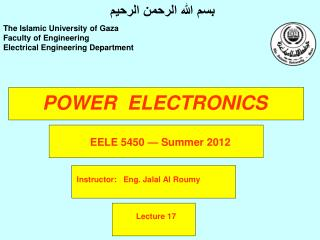DownloadDownload PresentationPOWER ELECTRONICS

# POWER ELECTRONICS

Download Presentation## POWER ELECTRONICS

- - - - - - - - - - - - - - - - - - - - - - - - - - - E N D - - - - - - - - - - - - - - - - - - - - - - - - - - -
##### Presentation Transcript

1. بسم الله الرحمن الرحيم The Islamic University of Gaza Faculty of Engineering Electrical Engineering Department POWER ELECTRONICS EELE 5450— Summer 2012 Instructor: Eng. Jalal Al Roumy Lecture 17

4. Chapter seven HALF-BRIDGE INVERTER WITH RESISTIVE LOAD AND CAPACITIVE ELEMENTS In the circuit shown, capacitors replace the centre-tapped battery, and a single battery acts as the d.c. source.

5. Chapter seven HALF-BRIDGE INVERTER WITH RESISTIVE LOAD AND CAPACITIVE ELEMENTS C1 and C2 are each charged to Vb/2 prior to switching. When Q1 is switched on, C1 capacitor voltage is applied across the load, load current flows and exponentially decays until Q1 is switched off.

6. Chapter seven HALF-BRIDGE INVERTER WITH RESISTIVE LOAD AND CAPACITIVE ELEMENTS Q2 is switched on to connect C2 as a reverse voltage across the load. Load current flows in reverse through the load, exponentially decaying until Q2 turns off and Q1 turns on to repeat the cycle.

7. Chapter seven HALF-BRIDGE INVERTER WITH RESISTIVE LOAD AND CAPACITIVE ELEMENTS Load voltage

8. Chapter seven HALF-BRIDGE INVERTER WITH RESISTIVE LOAD AND CAPACITIVE ELEMENTS Example Vb = 40V, C 1 - C 2 = 200μF, R1 = 10Ω and an inverter frequency of 100Hz. Assuming ideal switches, determine maximum and minimum values of load voltage, assuming the waveform is symmetrical.

9. Chapter seven HALF-BRIDGE INVERTER WITH RESISTIVE LOAD AND CAPACITIVE ELEMENTS Example Vb = 40V, C 1 - C 2 = 200μF, R1 = 10Ω and an inverter frequency of 100Hz. Assuming ideal switches, determine maximum and minimum values of load voltage, assuming the waveform is symmetrical.

10. Chapter seven

11. Chapter seven

12. Chapter seven HALF-BRIDGE WITH PURELY INDUCTIVE LOAD With resistive loads, at the half-cycle period Q1 is switched off and Q2 is switched on. The load current changes instantaneously from the maximum positive to the maximum negative value. With inductive loads, instantaneous reversal is impossible. In fact, if an inductive load was turned off with maximum current and there was no alternative path for the current to flow, the current collapse would be very rapid, and the induced voltage, e = L di/dt, would be very large. This voltage would appear across the transistor switch in reverse and could destroy it.

13. Chapter seven HALF-BRIDGE WITH PURELY INDUCTIVE LOAD To protect the transistor from this inductive surge, feedback diodes are connected in anti-parallel across the transistor to provide a path for the current to decay.

14. Chapter seven HALF-BRIDGE WITH PURELY INDUCTIVE LOAD When Q1 switches off at maximum positive current, the inductive voltage VL reverses its polarity, the voltage rises above Vb2 and forward biases D2, allowing the decay of current until load current zero, Then Q2 will start the flow of current in the negative direction.

15. Chapter seven HALF-BRIDGE WITH PURELY INDUCTIVE LOAD When negative current reaches its maximum value, Q2 switches off, VL reverses, rising above Vbl and forward biasing diode D1, until load current zero, Q1 will then allow current to grow in the positive direction and the cycle is repeated.

16. Chapter seven HALF-BRIDGE WITH PURELY INDUCTIVE LOAD

17. Chapter seven HALF-BRIDGE WITH PURELY INDUCTIVE LOAD

18. Chapter seven HALF-BRIDGE WITH PURELY INDUCTIVE LOAD

19. Chapter seven HALF-BRIDGE WITH PURELY INDUCTIVE LOAD

20. Chapter seven End of Lecture